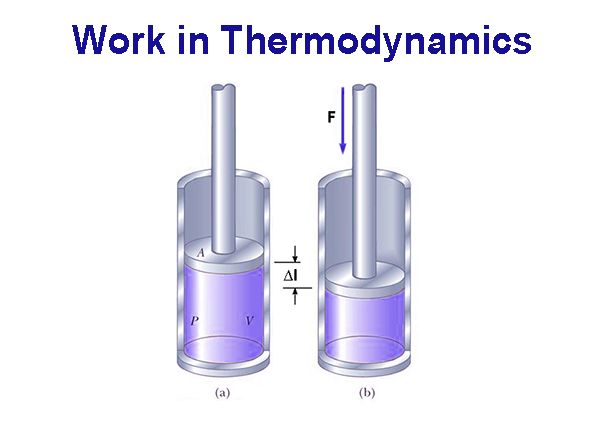# Work in Thermodynamics

In thermodynamics, work has a broader meaning. It includes mechanical work, electrical work, surface work etc. Mechanical work (w) is said to be done when an object is moved a distance ∆Ι by an applied force F.

Accordingly,

w = F x ∆l

If as a result of the application of the force F the object moves an infinitesimally small distance dl then the infinitesimally small work done dw would be given by

dw = F x dl

Unit of work:

The unit of force in the CGS units is dyne and that of length is centimeter. Work in the same unit is expressed in ergs.

So, dyne × cm = erg; erg is the work done when a force of 1 dyne acts and causes of displacement of 1 cm.

Since an erg of work is a small quantity, a larger unite, joule, is often used- 1 joule = 107 erg.

Characteristics of heat and work:

(a) Both appear at the boundary of the system

(b) Both cause changes in the system

(c) Both produce equivalent and opposite effects in the surroundings.